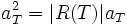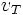Specht module

Definition

A Specht module is an indecomposable submodule of the algebra Failed to parse (unknown function "\QS"): \QS_n , or equivalently it is an irreducible representation of a symmetric group over the rational numbers. The symmetric group is a rational group, and hence the Specht modules are not only irreducible over$\Q$, they are also irreducible upon tensoring with$\C$.

Specht modules are explicitly constructed by means of Young tableaux, as the submodule generated by elements corresponding to various possible standard tableaux corresponding to a particular Young diagram (that is, shape of a tableau).

The fact that the Specht modules are irreducible can be deduced from the fact that the number of Specht modules is equal to the number of conjugacy classes, both being equal to the number of partitions of the integer$n$, along with the fact that the total number of irreducible representations over$\C$ also equials the number of conjugacy classes.

Construction

Young symmetrizers

For any Young tableau$T$with$n$ cells, define the group$R(T)$ (called the row group of the tableau) as the group of all permutations of the numbers which sends each row to itself. Similarly, define the column group$C(T)$ as the group of all permutations of the cells which send each column to itself. Now define the row and column symmetrizers as the following elements in the group ring:

Row symmetrizer:$a_T = \sum_{p \in R(T)} p$

Column symmetrizer:$b_T = \sum_{q \in C(T)} sgn(q) q$

where$sgn(q)$ denotes the sign of the permutation$q$.

The row symmetrizer is an eigenvector for left/right multiplication by any element of the row subgroup, with eigenvalue 1. The column symmetrizer is an eigenvector for left/right multiplication by any element of the column subgroup, with eigenvalue equal to the sign ofthat element.

Thus:$a_T^2 = |R(T)|a_T$

and:$b_T^2 = |C(T)|b_T$

Now define$M^\lambda$ to be the vector space with basis as the tabloids (a tabloid is an orbit of a Young tableau under the action of the row group). Since$S_n$ acts on the set of all tableaux, it acts on the set of tabloids, and thus$M_\lambda$ is a module over$S_n$.

Define$S^\lambda$ as the submodule of this spanned by the$v_T$s, where the$v_T$s are obtained by applying$b_T$ to the tabloids$T$.

Then, this$S_\lambda$ is the Specht module.

Dimension of the Specht module

We know that$M^\lambda$ is indexed by the number of tabloids of a given shape, viz the number of fillings of the Young diagram upto row equivalence. Some combinatorial lemmas show us that if we simply take the standard tableaux of shape$\lambda$, the$v_T$s for these standard tableaux is actually the whole of$S_\lambda$. Moreover, they are also linearly independent, which shows that the dimension of this module is$d_\lambda$ (the number of standard tableaux who shape is the given Young diagram).